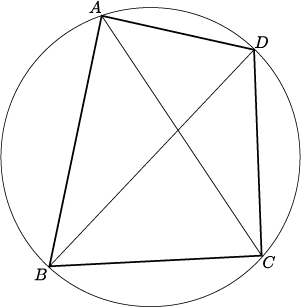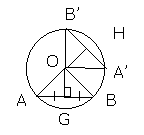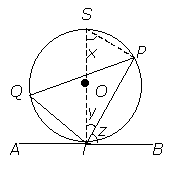Properties of Circles
PROPERTIES OF CIRCLES

Introduction

A circle is a simple, beautiful and symmetrical shape.
When a circle is rotated through any angle about its centre, its orientation remains the same.
When any straight line is drawn through its centre, it divides the circle into two identical semicircles.
The line is known as the diameter.
The common distance of the points of a circle from its centre is called radius.
The perimeter or length of the circle is also known as the circumference.Diameter
The diameter of a circle is the length of a line segment whose endpoints lie on the circle and which passes through the centre of the circle.
This is the largest distance between any two points on the circle.
The diameter of a circle is twice its radius.
In other words, two radius make one diameter.

Chords
A chord of a circle is a line segment whose two endpoints lie on the circle.
The diameter, passing through the circle's centre, is the largest chord in a circle.

Tangent
A tangent to a circle is a straight line that touches the circle at a single point.

Secant
A secant is an extended chord: a straight line cutting the circle at two points.Arc
An arc of a circle is any connected part of the circle's circumference.

Sector
A sector is a region bounded by two radius and an arc lying between the radius.

Segment
A segment is a region bounded by a chord and an arc lying between the chord's endpoints.__________________________________________________________________________________________________________________________

Angle Properties in a circle

Angles in the same segment are equal.

Proof:

AôB = 2x1 = 2x2 (Ð at centre= 2Ðat ⊙ce)
x1 = x2
ÐAPB = ÐAQB

Property can be abbreviated as Ðs in the same segment.Angles in a semicircle = 90°.∠B = 90°

Proof:

AôB = 2AĈB ( at centre = 2 at )
But AôB = 180°
AĈB = 90°Angle at the centre = twice the angle subtended by the same arc at the circumference.

Proof:
In the figure below, the angles are subtended by the minor arc AB. Since OA = OD (radii of circle), a = b (base angles of isos. triangle)
But angle AôE if the exterior angle of triangle AOD
AôE = 2a
Similarly, c = d (base angles of isos. triangle)
BôE = 2c
Hence, AôB = 2a + 2c = 2(a + c) = 2 angle ADB__________________________________________________________________________________________________________________

Angle Properties of a Cyclic Quadrilateral

Angles in opposite segments are supplementary.∠A + ∠C = 180° ; ∠B + ∠D = 180°∠A + ∠C = 180° ; ∠B + ∠D = 180°

Proof:
Let b = 50
2d = 360 - 100 = 260
d = 260/2 = 30
b + d = 30 + 50 = 180.

Property can be abbreviated as opp. Ðs of cyclic quad.

Exterior angle of a cyclic quadrilateral = the interior opposite angle.a° = b°

Proof:

b + d = 180°(opp. angles of cyclic quad.)
x + d = 180°(adj. angles on a str. l)
b + d = x + d
b = x
angle ABC = angle CDE

Property can be abbreviated as ext.Ð of a cyclic quad.
__________________________________________________________________________________________________________________________

Symmetrical Properties of Circle

Property 1:

A circle is symmetrical about every diameter. Hence any chord AB perpendicular to a diameter is bisected by the diameter.

Also, any chord bisected by a diameter is perpendicular to the diameter.

Proof:
Given a circle, centre O and a chord, AB, with a mid-point D, we are required to show that OĈB = 90°.
Join OA and OB. In triangle OAC and OBC,
OA = OB (radii of circle)
AC = BC (given)
OC is common.

Triangle OCD is congruent to triangle OBC (SSS property)

OĈA = OĈB.

Since these are adjacent angles on a straight line, OĈA = OĈB = 90°Property 2:

In equal circles or in the same circle, equal chords are equidistant from the centre. Chords which are equidistant from the centre are equal.

Proof:

In the figure, triangle OAB is rotated through an angle AOA' to triangle OA'B' about O.
Since rotation preserves shape and size, AB = A'B' and OG = OH.__________________________________________________________________________________________________________________________

Alternate Segment Theorem

The alternate segment theorem states that an angle between a tangent and a chord through the point of contact is equal to the angle in the alternate segment. Thus, ÐPTB = ÐPQT.

Proof:
ÐSPT = 90° (rt. Ð in a semicircle)
Ðx + Ðy = 90° ( Ð sum of ∆)
Ðy + Ðz = 90° ( tan rad.)
Ðx = Ðz
ÐPTB = ÐPST
ÐPST = ÐPQT ( Ðs in same segment)
ÐPTB = ÐPQT__________________________________________________________________________________________________________________________

The Singapore Flyer is a symmetrical obeservatory wheel. It is also the largest in the world. For the Singapore Flyer to spin, the wheel have to be symmetrical. If it isn't symmetrical, it would not be able to turn and thus, people might not be able to enjoy the nice scenery of Singapore.

Group Member(s).
Jason Chua Wei Xiang (21)

Loo Wen Hao (30)

Lyman Ng Quan Li (31)

Nicholas Lee Jia Ming (35)

Class.
3D

Topic.
Properties of Circles

Games.

Bibliography.
Soon May Ling, Dr Spario Soon (2008)
Comprehensive Notes on 'O' Level Mathematics (Paper 4016)
Learning Interactive Pte Ltd (Singapore)

Chow Wai Keung, Esther Ng Yoon Cheng,
Prof Ling San (2007)
discovering MATHEMATICS 3A
Star Publishing Pte Ltd (Singapore)

http://en.wikipedia.org/wiki/Circle

http://library.thinkquest.org/C0110248/geometry
/circlesy.htm

URL.